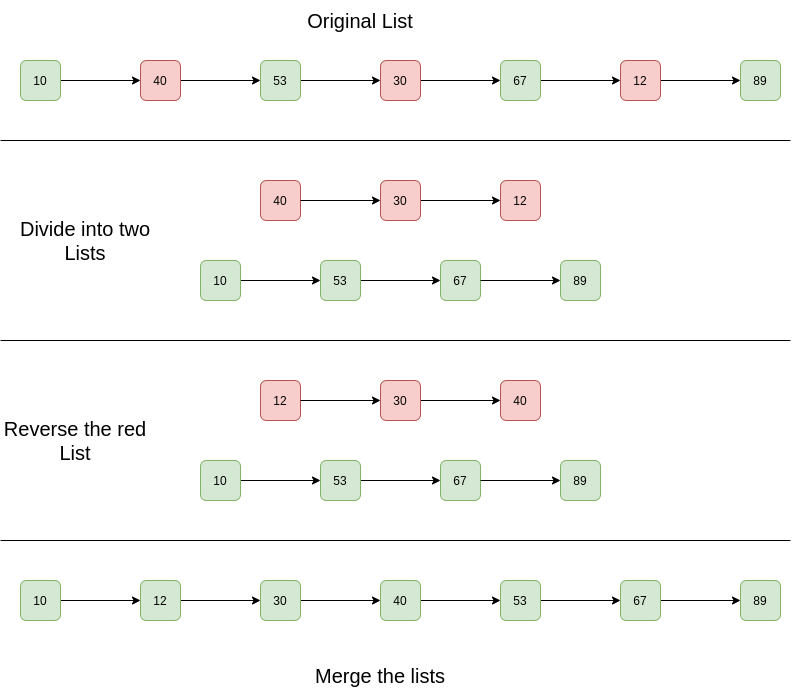GeeksforGeeks App
Open AppBrowser
Continue

# Python Program For Sorting A Linked List That Is Sorted Alternating Ascending And Descending Orders

Given a Linked List. The Linked List is in alternating ascending and descending orders. Sort the list efficiently.

Example:

```Input List: 10 -> 40 -> 53 -> 30 -> 67 -> 12 -> 89 -> NULL
Output List: 10 -> 12 -> 30 -> 40 -> 53 -> 67 -> 89 -> NULL

Input List: 1 -> 4 -> 3 -> 2 -> 5 -> NULL
Output List: 1 -> 2 -> 3 -> 4 -> 5 -> NULL```

Simple Solution:
Approach: The basic idea is to apply to merge sort on the linked list.
Complexity Analysis:

• Time Complexity: The merge sort of linked list takes O(n log n) time. In the merge sort tree, the height is log n. Sorting each level will take O(n) time. So time complexity is O(n log n).
• Auxiliary Space: O(n log n), In the merge sort tree the height is log n. Storing each level will take O(n) space. So space complexity is O(n log n).

Efficient Solution:
Approach:

1. Separate two lists.
2. Reverse the one with descending order
3. Merge both lists.

Diagram:Below are the implementations of the above algorithm:

## Python

 `# Python program to sort a linked list ``# that is alternatively sorted in ``# increasing and decreasing order``class` `LinkedList(``object``):``    ``def` `__init__(``self``):``        ``self``.head ``=` `None`` ` `    ``# Linked list Node``    ``class` `Node(``object``):``        ``def` `__init__(``self``, d):``            ``self``.data ``=` `d``            ``self``.``next` `=` `None`` ` `    ``def` `newNode(``self``, key):``        ``return` `self``.Node(key)`` ` `    ``# This is the main function that sorts``    ``# the linked list.``    ``def` `sort(``self``):``        ``# Create two dummy nodes and ``        ``# initialize as``        ``# heads of linked lists``        ``Ahead ``=` `self``.Node(``0``)``        ``Dhead ``=` `self``.Node(``0``)`` ` `        ``# Split the list into lists``        ``self``.splitList(Ahead, Dhead)``        ``Ahead ``=` `Ahead.``next``        ``Dhead ``=` `Dhead.``next`` ` `        ``# Reverse the descending list``        ``Dhead ``=` `self``.reverseList(Dhead)`` ` `        ``# Merge the 2 linked lists``        ``self``.head ``=` `self``.mergeList(Ahead, ``                                   ``Dhead)`` ` `    ``# Function to reverse the linked list``    ``def` `reverseList(``self``, Dhead):``        ``current ``=` `Dhead``        ``prev ``=` `None``        ``while` `current !``=` `None``:``            ``self``._next ``=` `current.``next``            ``current.``next` `=` `prev``            ``prev ``=` `current``            ``current ``=` `self``._next``        ``Dhead ``=` `prev``        ``return` `Dhead`` ` `    ``# Function to print linked list``    ``def` `printList(``self``):``        ``temp ``=` `self``.head``        ``while` `temp !``=` `None``:``            ``print` `temp.data,``            ``temp ``=` `temp.``next``        ``print` `''`` ` `    ``# A utility function to merge two ``    ``# sorted linked lists``    ``def` `mergeList(``self``, head1, head2):``        ``# Base cases``        ``if` `head1 ``=``=` `None``:``            ``return` `head2``        ``if` `head2 ``=``=` `None``:``            ``return` `head1``        ``temp ``=` `None``        ``if` `head1.data < head2.data:``            ``temp ``=` `head1``            ``head1.``next` `=` `self``.mergeList(head1.``next``, ``                                        ``head2)``        ``else``:``            ``temp ``=` `head2``            ``head2.``next` `=` `self``.mergeList(head1, ``                                        ``head2.``next``)``        ``return` `temp`` ` `    ``# This function alternatively splits a ``    ``# linked list with head as head into two:``    ``# For example, 10->20->30->15->40->7 is ``    ``# splitted into 10->30->40 and 20->15->7``    ``# "Ahead" is reference to head of ascending ``    ``# linked list``    ``# "Dhead" is reference to head of descending ``    ``# linked list``    ``def` `splitList(``self``, Ahead, Dhead):``        ``ascn ``=` `Ahead``        ``dscn ``=` `Dhead``        ``curr ``=` `self``.head`` ` `        ``# Link alternate nodes``        ``while` `curr !``=` `None``:`` ` `            ``# Link alternate nodes in ascending ``            ``# order``            ``ascn.``next` `=` `curr``            ``ascn ``=` `ascn.``next``            ``curr ``=` `curr.``next``            ``if` `curr !``=` `None``:``                ``dscn.``next` `=` `curr``                ``dscn ``=` `dscn.``next``                ``curr ``=` `curr.``next``        ``ascn.``next` `=` `None``        ``dscn.``next` `=` `None`` ` `# Driver code``llist ``=` `LinkedList()``llist.head ``=` `llist.newNode(``10``)``llist.head.``next` `=` `llist.newNode(``40``)``llist.head.``next``.``next` `=` `llist.newNode(``53``)``llist.head.``next``.``next``.``next` `=` `llist.newNode(``30``)``llist.head.``next``.``next``.``next``.``next` `=` `llist.newNode(``67``)``llist.head.``next``.``next``.``next``.``next``.``next` `=` `llist.newNode(``12``)``llist.head.``next``.``next``.``next``.``next``.``next``.``next` `=` `llist.newNode(``89``)`` ` `print` `'Given linked list'``llist.printList()`` ` `llist.sort()`` ` `print` `'Sorted linked list'``llist.printList()``# This code is contributed by BHAVYA JAIN`

Output:

```Given Linked List is
10 40 53 30 67 12 89